GATE  >  Mining Engineering - MN 2014 GATE Paper (Practice Test)

# Mining Engineering - MN 2014 GATE Paper (Practice Test) - GATE

Test Description

## 65 Questions MCQ Test GATE Past Year Papers for Practice (All Branches) - Mining Engineering - MN 2014 GATE Paper (Practice Test)

Mining Engineering - MN 2014 GATE Paper (Practice Test) for GATE 2023 is part of GATE Past Year Papers for Practice (All Branches) preparation. The Mining Engineering - MN 2014 GATE Paper (Practice Test) questions and answers have been prepared according to the GATE exam syllabus.The Mining Engineering - MN 2014 GATE Paper (Practice Test) MCQs are made for GATE 2023 Exam. Find important definitions, questions, notes, meanings, examples, exercises, MCQs and online tests for Mining Engineering - MN 2014 GATE Paper (Practice Test) below.
Solutions of Mining Engineering - MN 2014 GATE Paper (Practice Test) questions in English are available as part of our GATE Past Year Papers for Practice (All Branches) for GATE & Mining Engineering - MN 2014 GATE Paper (Practice Test) solutions in Hindi for GATE Past Year Papers for Practice (All Branches) course. Download more important topics, notes, lectures and mock test series for GATE Exam by signing up for free. Attempt Mining Engineering - MN 2014 GATE Paper (Practice Test) | 65 questions in 180 minutes | Mock test for GATE preparation | Free important questions MCQ to study GATE Past Year Papers for Practice (All Branches) for GATE Exam | Download free PDF with solutions
 1 Crore+ students have signed up on EduRev. Have you?
Mining Engineering - MN 2014 GATE Paper (Practice Test) - Question 1

### Q. 1 – Q. 5 carry one mark each. Q. Choose the most appropriate word from the options given below to complete the following sentence. A person suffering from Alzheimer’s disease short-term memory loss.

Mining Engineering - MN 2014 GATE Paper (Practice Test) - Question 2

### Choose the most appropriate word from the options given below to complete the following sentence. ____________ is the key to their happiness; they are satisfied with what they have

Mining Engineering - MN 2014 GATE Paper (Practice Test) - Question 3

### Which of the following options is the closest in meaning to the sentence below? “As a woman, I have no country.”

*Answer can only contain numeric values
Mining Engineering - MN 2014 GATE Paper (Practice Test) - Question 4

In any given year, the probability of an earthquake greater than Magnitude 6 occurring in the
Garhwal Himalayas is 0.04. The average time between successive occurrences of such earthquakes
is ____ years.

(Important : you should answer only the numeric value)

Mining Engineering - MN 2014 GATE Paper (Practice Test) - Question 5

The population of a new city is 5 million and is growing at 20% annually. How many years would
it take to double at this growth rate?

Mining Engineering - MN 2014 GATE Paper (Practice Test) - Question 6

Q. 6 – Q. 10 carry two marks each.

Q.

In a group of four children, Som is younger to Riaz. Shiv is elder to Ansu. Ansu is youngest in the
group. Which of the following statements is/are required to find the eldest child in the group?
Statements
1. Shiv is younger to Riaz.
2. Shiv is elder to Som.

Mining Engineering - MN 2014 GATE Paper (Practice Test) - Question 7

Moving into a world of big data will require us to change our thinking about the merits of
exactitude. To apply the conventional mindset of measurement to the digital, connected world of
the twenty-first century is to miss a crucial point. As mentioned earlier, the obsession with
exactness is an artefact of the information-deprived analog era. When data was sparse, every data
point was critical, and thus great care was taken to avoid letting any point bias the analysis.
From “BIG DATA” Viktor Mayer-Schonberger and Kenneth Cukier
The main point of the paragraph is:

Mining Engineering - MN 2014 GATE Paper (Practice Test) - Question 8

The total exports and revenues from the exports of a country are given in the two pie charts below.
The pie chart for exports shows the quantity of each item as a percentage of the total quantity of
exports. The pie chart for the revenues shows the percentage of the total revenue generated through
export of each item. The total quantity of exports of all the items is 5 lakh tonnes and the total
revenues are 250 crore rupees. What is the ratio of the revenue generated through export of Item 1
per kilogram to the revenue generated through export of Item 4 per kilogram?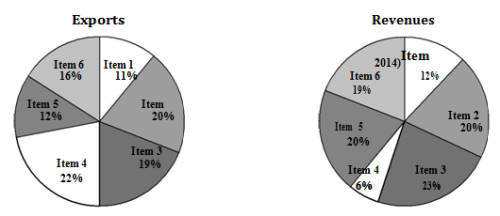Mining Engineering - MN 2014 GATE Paper (Practice Test) - Question 9

X is 1 km northeast of Y. Y is 1 km southeast of Z. W is 1 km west of Z. P is 1 km south of W. Q is
1 km east of P. What is the distance between X and Q in km?

*Answer can only contain numeric values
Mining Engineering - MN 2014 GATE Paper (Practice Test) - Question 10

10% of the population in a town is HIV+. A new diagnostic kit for HIV detection is available; this
kit correctly identifies HIV+ individuals 95% of the time, and HIV individuals 89% of the time. A
particular patient is tested using this kit and is found to be positive. The probability that the
individual is actually positive is _______

Mining Engineering - MN 2014 GATE Paper (Practice Test) - Question 11

Q. 11 – Q. 25 carry one mark each.

Q.

A block of weight 100 kN rests on a floor as shown in the figure. The coefficient of static friction
between the block and the floor is 0.5. A force of 45 kN is applied horizontally on the block. The
static frictional force in kN is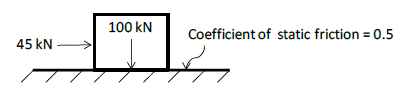Mining Engineering - MN 2014 GATE Paper (Practice Test) - Question 12

A spring of constant stiffness k is stretched from point A to point B (displacement u in the figure)
by a force F. The potential energy of the spring is expressed by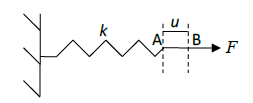Mining Engineering - MN 2014 GATE Paper (Practice Test) - Question 13

If σs is the induced stress and σi is the insitu stress at a point below ground, the ‘stress
concentration’ at that point is

*Answer can only contain numeric values
Mining Engineering - MN 2014 GATE Paper (Practice Test) - Question 14

The components of state of stress at a point in x−y plane are given as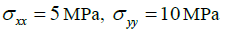and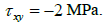The sum of the principal stresses acting on the x−y plane in MPa is _______

(Important : you should answer only the numeric value)

Mining Engineering - MN 2014 GATE Paper (Practice Test) - Question 15

The andgle 5o 15' 25" is expressed in hours, minutes and seconds as

Mining Engineering - MN 2014 GATE Paper (Practice Test) - Question 16

A circular curve has a radius of 200 m and deflection angle of 650. The length of the curve in m is

*Answer can only contain numeric values
Mining Engineering - MN 2014 GATE Paper (Practice Test) - Question 17

The weight strength of ANFO of specific gravity 0.8 is 912 kcal/kg. The weight strength of an
emulsion explosive of specific gravity 1.2 is 850 kcal/kg. Bulk strength of the emulsion explosive
relative to ANFO in percentage is _________

Mining Engineering - MN 2014 GATE Paper (Practice Test) - Question 18

In a cut-and-fill stope, the main purpose of back filling is to

Mining Engineering - MN 2014 GATE Paper (Practice Test) - Question 19

Bypass valve in a compressed oxygen type self-contained breathing apparatus is meant to

Mining Engineering - MN 2014 GATE Paper (Practice Test) - Question 20

Given S is the setting load and Y is the yield load of a hydraulic prop, the correct relationship is

Mining Engineering - MN 2014 GATE Paper (Practice Test) - Question 21

Solution of the differential equation dy/dx= ky follows exponential decay ( where k is a constant) for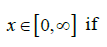*Answer can only contain numeric values
Mining Engineering - MN 2014 GATE Paper (Practice Test) - Question 22

The value of k for which the vectors a = 2i - 3j and b = k i + 4j are orthogonal to each other is _______

(Important : you should answer only the numeric value)

Mining Engineering - MN 2014 GATE Paper (Practice Test) - Question 23

Which one of the following is the most likely mode of slope failure for waste dump

*Answer can only contain numeric values
Mining Engineering - MN 2014 GATE Paper (Practice Test) - Question 24

The occurrence of head in a single toss of an unbiased coin is given by a random variable X. The
variance of X is _______________

(Important : you should answer only the numeric value)

Mining Engineering - MN 2014 GATE Paper (Practice Test) - Question 25

The divergence of the vector v = (x + y)(−yi + xj) is

Mining Engineering - MN 2014 GATE Paper (Practice Test) - Question 26

The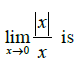Mining Engineering - MN 2014 GATE Paper (Practice Test) - Question 27

For Indian coal mines, the ‘maximum allowable concentration’ of respirable dust containing 7.5%
free silica in mg/m3 is

Mining Engineering - MN 2014 GATE Paper (Practice Test) - Question 28

Given ???? is the thermal conductivity, ?? is density and ?? is specific heat of a rock sample, the thermal
diffusivity of the rock sample is

Mining Engineering - MN 2014 GATE Paper (Practice Test) - Question 29

Cyclone, bag filter and scrubber can be used for control of

Mining Engineering - MN 2014 GATE Paper (Practice Test) - Question 30

A mine waste dump of pH 5.2 can be neutralized by adding

*Answer can only contain numeric values
Mining Engineering - MN 2014 GATE Paper (Practice Test) - Question 31

A flat coal seam of thickness (t) 3 m is excavated and broken roof rock has completely filled the
space created due to extraction as shown in the figure. If the bulking factor of roof rock is 1.2, the
caving height (H) in m is ______________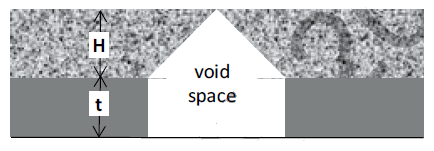(Important : you should answer only the numeric value)

*Answer can only contain numeric values
Mining Engineering - MN 2014 GATE Paper (Practice Test) - Question 32

A piece of coal sample weighs 10 kg in air and 2 kg when immersed in water. The specific gravity
of the coal sample is ______________________

(Important : you should answer only the numeric value)

Mining Engineering - MN 2014 GATE Paper (Practice Test) - Question 33

In a borehole log of 1.2 m in length, recovery of rock cores in cm is given below
20, 8, 15, 8, 8, 4, 3, 9, 10, 1, 5, 10
The RQD in percentage is

*Answer can only contain numeric values
Mining Engineering - MN 2014 GATE Paper (Practice Test) - Question 34

An underground coal mine panel produces 520 tonnes per day deploying 220, 200 and 192 persons
in three shifts. As per CMR 1957, the minimum quantity of air in m3/min to be delivered at the last
ventilation connection of the panel is ______________

(Important : you should answer only the numeric value)

Mining Engineering - MN 2014 GATE Paper (Practice Test) - Question 35

In a PERT network, the activities on the critical path are a, b and c. The standard deviations of the
durations of these activities are 2, 2 and 1 respectively. The variance of the project duration is

Mining Engineering - MN 2014 GATE Paper (Practice Test) - Question 36

Q. 36 – Q. 65 carry two marks each.

Q

A particle P is in equilibrium as shown in the figure. The magnitude in kN and the orientation θ in
degrees of the force F respectively are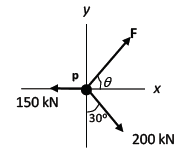*Answer can only contain numeric values
Mining Engineering - MN 2014 GATE Paper (Practice Test) - Question 37

A distributed load of 4 kN/m acts on a beam of 6 m length supported by a hinge and a roller as
shown in the figure. The distance in m of the point of zero shear in the beam from the point A is __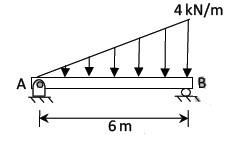*Answer can only contain numeric values
Mining Engineering - MN 2014 GATE Paper (Practice Test) - Question 38

A dry rock sample of diameter 50 mm and length 100 mm weighs 300 g. After saturating in brine
solution of specific gravity 1.05, its weight increased to 330 g. The porosity of the rock sample in
percentage is __________

Mining Engineering - MN 2014 GATE Paper (Practice Test) - Question 39

A joint plane of length L and dip δ intersects the toe of a slope as shown in the figure. The weight
of the shaded block is W. Uniform water pressure P acts normal to the joint plane. If the cohesion
and angle of internal friction of the joint surface are c and φ respectively, then the expression for
‘safety factor’ of the shaded block is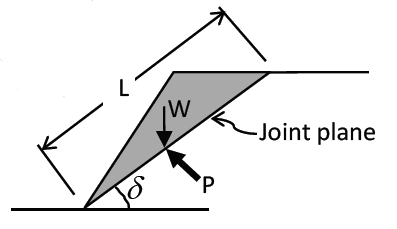Mining Engineering - MN 2014 GATE Paper (Practice Test) - Question 40

The lengths and standard errors of three sections AB, BC, and CD of a straight line AD are given
below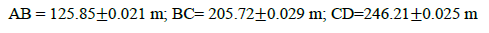The standard error in total length AD in m is

Mining Engineering - MN 2014 GATE Paper (Practice Test) - Question 41

The bearing of side AB of a regular hexagon ABCDEF is ??50010′ ?? . If the station C is easterly
from the station B, the whole circle bearing of the side BC is

Mining Engineering - MN 2014 GATE Paper (Practice Test) - Question 42

In a room-and-pillar stope, bench blasting is conducted using ANFO having density of 800 kg/m3.
The specific gravity of rock is 2.5, hole diameter is 100 mm and spacing to burden ratio is 1.3. The
charge length of each blast hole is 80% of the hole length. For a desired powder factor of 0.48
kg/tonne, the spacing and burden of the blast pattern in m respectively are

Mining Engineering - MN 2014 GATE Paper (Practice Test) - Question 43

Match the following for ore handling operations in an underground metal mine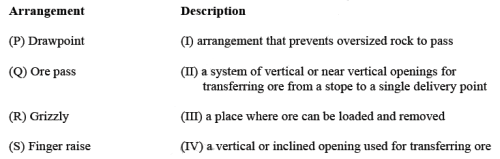Mining Engineering - MN 2014 GATE Paper (Practice Test) - Question 44

The following characteristic curves (P, Q, R, S) pertain to rotary drilling in rock.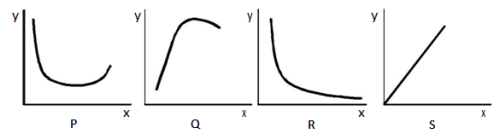Title of the curve
I: Torque versus RPM
II: Rate of penetration versus uniaxial compressive strength of rock
III: Rate of penetration versus weight on bit
IV: Specific energy versus weight on bit
Match the curves with their titles

*Answer can only contain numeric values
Mining Engineering - MN 2014 GATE Paper (Practice Test) - Question 45

The height H of a drawpoint in a sublevel caving stope is 3.0 m. If the angle of repose (??) of
broken ore is 350, the digging depth y of the loader as shown in the figure in m is _______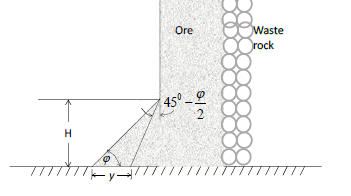*Answer can only contain numeric values
Mining Engineering - MN 2014 GATE Paper (Practice Test) - Question 46

For an explosives company, the probability of producing a defective detonator is 0.02. The
probability that a lot of 50 detonators produced by the company contains at most 2 defective
detonators is __________

Mining Engineering - MN 2014 GATE Paper (Practice Test) - Question 47

The area enclosed by the curves y = x2 and y = x3  for x∈[0,∞] is

Mining Engineering - MN 2014 GATE Paper (Practice Test) - Question 48

The value of a, for which the function below is continuous at x = 1 is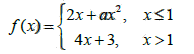Mining Engineering - MN 2014 GATE Paper (Practice Test) - Question 49

The sum of the infinite series a + ar + ar2 + ar3 +...+ arn−1 +..... for r <1 is

Mining Engineering - MN 2014 GATE Paper (Practice Test) - Question 50

A centrifugal pump has a discharge rate of 2000 L of water per min against a total head of 200 m. If
the pump efficiency is 75%, the input power to the pump in kW is

Mining Engineering - MN 2014 GATE Paper (Practice Test) - Question 51

A dragline is required to remove 3,00,000 m3 of rock per month on the bank volume basis.
Consider the following data for the dragline operation.
Effective working hours per month = 450
Bucket fill factor = 0.8
Cycle time = 65 s
Swell factor of the rock = 1.25
The minimum bucket capacity of the dragline in m3 is

Mining Engineering - MN 2014 GATE Paper (Practice Test) - Question 52

A direct rope haulage pulls 8 tubs loaded with coal through an incline of length 500 m having an
inclination of 1 in 6. Consider the following additional data.
Capacity of tub = 1.0 tonne
Tare weight of tub = 500 kg
Hauling speed = 9 km per hour
Coefficient of friction between wheel and rail = 1/60
Coefficient of friction between rope and drum = 1/10
Mass of rope per meter = 1.5 kg
The minimum power required to haul the tubs in kW is

*Answer can only contain numeric values
Mining Engineering - MN 2014 GATE Paper (Practice Test) - Question 53

A coal mine receives two bids for purchase of a new dragline. The first bid quotes Rs. 150 crore as
a price to be paid in full on delivery. The second bid quotes Rs. 180 crore as a price payable at the
end of the third year after delivery. If the discount rate is 12%, the difference in NPV between the
first and second bids in crore of rupees is __________

(Important : you should answer only the numeric value)

Mining Engineering - MN 2014 GATE Paper (Practice Test) - Question 54

Match the following in the context of underground mine environment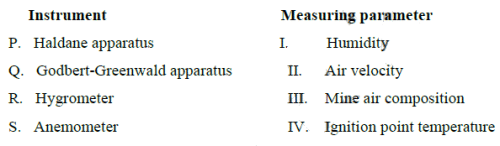*Answer can only contain numeric values
Mining Engineering - MN 2014 GATE Paper (Practice Test) - Question 55

A mine airway having cross-section of 2.2 m × 2.2 m and length 500 m contains a bend. Given that
the airway friction factor is 0.01 Ns2m−4, shock loss factor for the bend is 0.07 and density of air is
1.2 kg/m3, the equivalent length of the airway in m is __________

*Answer can only contain numeric values
Mining Engineering - MN 2014 GATE Paper (Practice Test) - Question 56

In order to estimate the NVP in a mine, measurements are made at the main fan as shown below.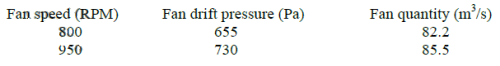The NVP in Pa is ___________

*Answer can only contain numeric values
Mining Engineering - MN 2014 GATE Paper (Practice Test) - Question 57

The resistances of two splits A and B are 0.35 Ns2m−8 and 0.05 Ns2m−8 respectively. The combined
resistance of the shafts and trunk airways is 0.4 Ns2m−8. A booster fan is planned to be installed in
split A to increase the quantity flowing through it. Assuming that the surface fan continues to
operate at a constant pressure of 1000 Pa, the critical pressure of the booster fan in Pa is _______

*Answer can only contain numeric values
Mining Engineering - MN 2014 GATE Paper (Practice Test) - Question 58

A pitot tube is inserted in a ventilation duct with the nose facing the air flow. A vertical U-tube
manometer filled with alcohol (specific gravity 0.8) has been used for pressure measurements such
that 10.2 mm is read as the total pressure and 8.8 mm as the static pressure. Given the density of air
to be 1.2 kg/m3, the air velocity at the nose of the pitot tube in m/s is ____________

*Answer can only contain numeric values
Mining Engineering - MN 2014 GATE Paper (Practice Test) - Question 59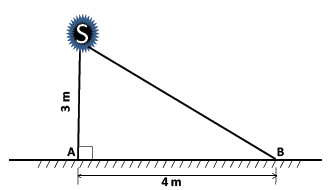An illumination source S shown in the figure emits light equally in all directions. At a point A on
the floor, the illuminance is 5.0 lux. The illuminance at point B on the floor in lux is ________

Mining Engineering - MN 2014 GATE Paper (Practice Test) - Question 60

Two machines A and B while operating simultaneously produce a sound pressure level of 85 dBA
at a point. When the machine A stops, the sound pressure level at that point reduces to 80 dBA. The
sound pressure level at the same point due to machine A operating alone in dBA is

Mining Engineering - MN 2014 GATE Paper (Practice Test) - Question 61

A waste water effluent has BOD5 of 80 mg/L and the reaction rate constant is 0.16 per day. The
ultimate BOD in mg/L is

Mining Engineering - MN 2014 GATE Paper (Practice Test) - Question 62

A series of tri-axial compression tests conducted on sandstone samples reveal the following
relationship between major and minor principal stresses
??1 = 50 + 3??3 [stresses are in MPa]
The cohesion in MPa and angle of internal friction in degrees of sandstone respectively are

*Answer can only contain numeric values
Mining Engineering - MN 2014 GATE Paper (Practice Test) - Question 63

Six detonators each having resistance of 1.5 ohm are connected in parallel. A 15 V exploder is
connected to the detonators by two single-core cables of resistance 3 ohm each. The current in the
circuit in Ampere is __________

*Answer can only contain numeric values
Mining Engineering - MN 2014 GATE Paper (Practice Test) - Question 64

The failure and the repair rates of a shovel are 0.06 hr−1 and 0.04 hr−1 respectively. The availability
of the shovel in percentage is ____________

(Important : you should answer only the numeric value)

*Answer can only contain numeric values
Mining Engineering - MN 2014 GATE Paper (Practice Test) - Question 65

The individual reliability values of four sub-systems are given in the figure below. The reliability of
the system is _________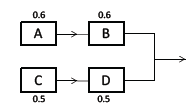## GATE Past Year Papers for Practice (All Branches)

407 docs|127 tests
Information about Mining Engineering - MN 2014 GATE Paper (Practice Test) Page
In this test you can find the Exam questions for Mining Engineering - MN 2014 GATE Paper (Practice Test) solved & explained in the simplest way possible. Besides giving Questions and answers for Mining Engineering - MN 2014 GATE Paper (Practice Test), EduRev gives you an ample number of Online tests for practice

## GATE Past Year Papers for Practice (All Branches)

407 docs|127 tests(Scan QR code)